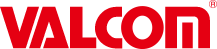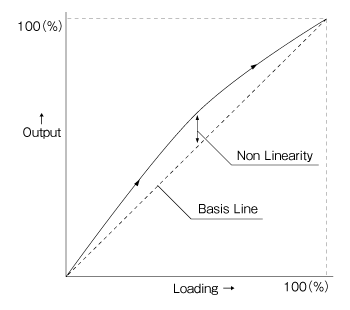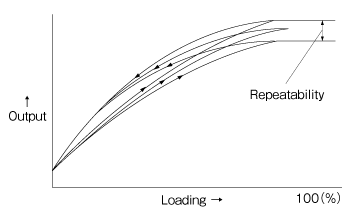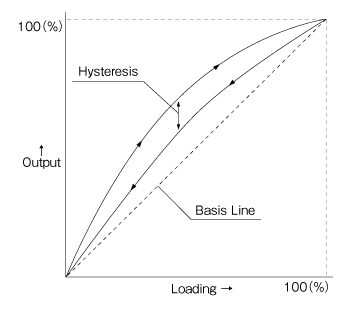Specialized manufacturer of Digital Pressure Meters and Load Cells

### Glossary on this Website

Rated Capacity(R.C.)
The maximum load that can be measured while meeting the specification. Also referred to as the rated load.
Rated Output(R.O.)
The difference after subtracting the output with no load from the rated load output. Usually expressed in mV/V, mA (output per 1 V of applied voltage) or as an equivalent strain.
Full Scale(F.S.)
Maximum measurable value.
Zero Balance
Output value when no load is applied. Usually expressed as the percentage of rated output, in the unit of mV/V or the value converted in terms of strain.
Nonlinearity
Output with increasing load and the maximum deviation for the reference line connecting the output with no load and the output with the rated load. Expressed as the percentage of the rated output.Repeatability
The maximum difference in output when the same load is applied repeatedly under the same condition. Usually measured with the rated load. Expressed as the percentage of average of rated output.Hysteresis
The maximum of difference in the outputs from the same test where load is increased to and decreased from the rated value in a reciprocating manner. Expressed as the percentage of the rated output.Excitation, Recommended
The voltage that is most suitable to use.
Excitation, Maximum
The maximum voltage that can be applied without changing the characteristics.
Input Terminal Resistance
The resistance to be measured between open input terminals with no load.
Output Terminal Resistance
The resistance to be measured between open output terminals with no load.
Input&Output Resistance
The resistance value seen from the input and output terminals. Used only when describing nominal values of input/output resistances.
Insulation Resistance
The direct current resistance between the transducer circuit and main body.
Compensated Temperature Range
The range of temperature compensated so that the influence of temperature on the output or zero point may be kept to within the specified limit.
Safe Temperature Range
The range of use that will not change the characteristics of transducer permanently.
Load of range beyond the rated capacity and outside of specification, but will not change the characteristics permanently. Expressed as the percentage of the rated capacity.
The maximum load that can be applied without causing structural damage.
Temperature Effect on Zero Balance
Changes in the zero balance caused by the changes in the ambient temperature in no-load condition. Usually the variation per 10°C is expressed as percentage of the rated capacity.
Changes in the output of rated load caused by the changes in the ambient temperature. Usually the variation per 10°C is expressed as percentage of the rated output.
Fastening Torque, Recomended
Range of fastening torque that is most suitable for use.
Fastening Torque, Maximum
The maximum allowable torque for fastening that can be applied without causing any damage to the structure.
Deflection at R.C.
Deflection of load cell when rated load is applied as seen from the position with no load.

### Note

Output shown in voltage and in strain

mV/V used to in output voltage of the transducer shows the output voltage (mV) per bridge voltage (1 V).
The relation between this voltage and strain (× 10−6) is as follows: 1 mV/V = 2000 × 10−6